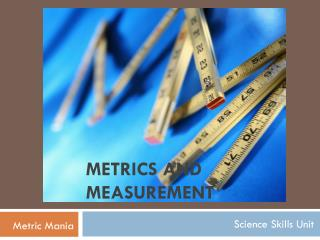DownloadDownload PresentationMetrics and Measurement

# Metrics and Measurement

Télécharger la présentation## Metrics and Measurement

- - - - - - - - - - - - - - - - - - - - - - - - - - - E N D - - - - - - - - - - - - - - - - - - - - - - - - - - -
##### Presentation Transcript

1. Metrics and Measurement Science Skills Unit Metric Mania

2. DENSITY Density • Definition: Mass per unit volume of an object. • Units: g/ml or g/cm3 • Tools Used: Calculator to divide two measurements. • Equation: density = mass / volume

3. DENSITY Find the density of the block on the left. The picture below is of the beams of a triple-beam balance after the block was placed on it. 1 cm 1 cm Volume: 2cm x 1cm x 1cm Volume = 2 cm3 2 cm Mass: 20g + 200g + 2g Mass = 222g Density: 222 g / 2 cm3 Density = 111 g/cm3

4. DENSITY Find the density of the marble below. Find the volume of the marble using the picture to the left of the water measurements before and after the marble was dropped in it. The graduated cylinders have measurements in milliliters. Volume: 25 ml – 15 ml Volume = 10 ml

5. DENSITY Find the density of the marble below. Find the mass of the marble using the picture above of the beams of a triple-beam balance after the marble was placed on it. Mass: 50g + 0g + 5g Mass = 55g Mass of Block: 55 grams Volume of Block: 10 ml Density of Block: 55g / 10ml = 5.5 g/ml